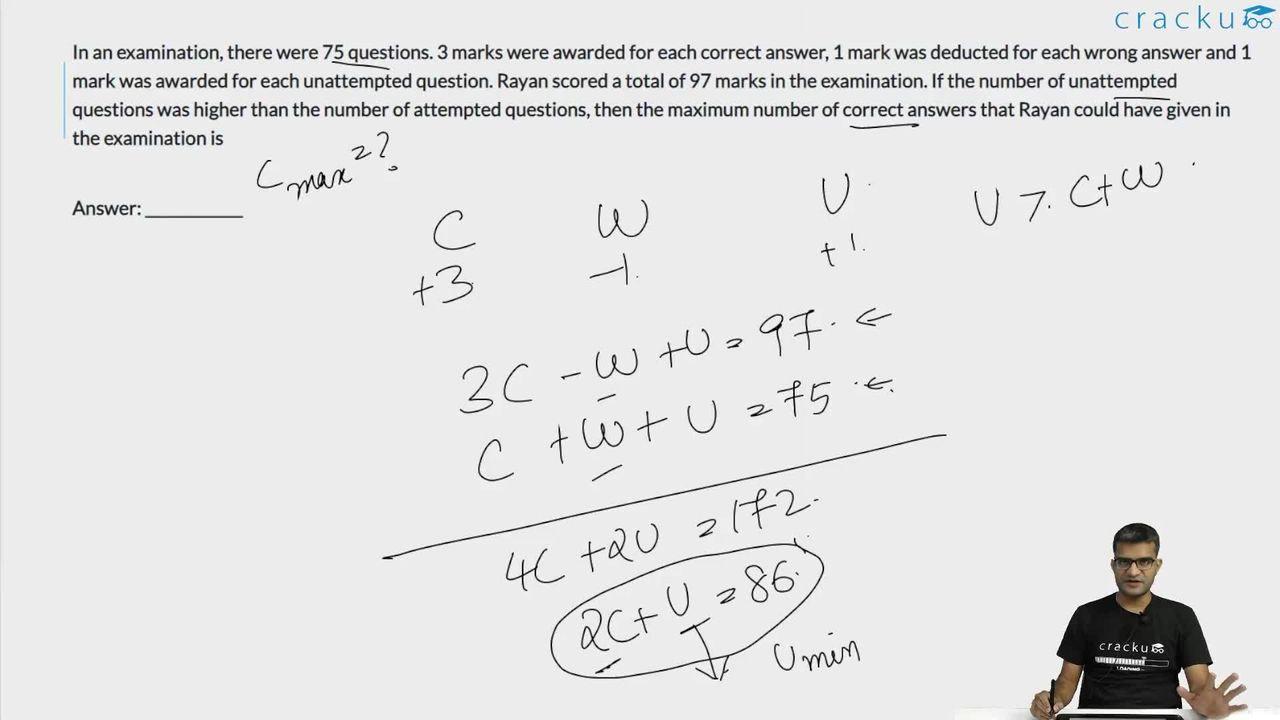Question 54

# In an examination, there were 75 questions. 3 marks were awarded for each correct answer, 1 mark was deducted for each wrong answer and 1 mark was awarded for each unattempted question. Rayan scored a total of 97 marks in the examination. If the number of unattempted questions was higher than the number of attempted questions, then the maximum number of correct answers that Rayan could have given in the examination is

Solution

Let the number of questions attempted be x+y out of which x are correct and y are incorrect and the number of questions unattempted be z.

It is given,

x + y + z = 75 ...... (1)

3x - y + z = 97 ...... (2)

(2)-(1) -> x - y = 11

(1)+(2) -> 2x + z = 86

z > x + y

z > 75 - z

z > 37.5

Minimum possible value of z is 38

2x + 38 = 86

2x = 48

x = 24

The maximum number of correct answers is 24.

### View Video Solution• All Quant CAT Formulas and shortcuts PDF
• 30+ CAT previous papers with solutions PDF

##### Srishti Soni

1 month, 2 weeks ago

Kuch bhi

##### Vikrant Agrawal

7 months, 2 weeks ago

No interested